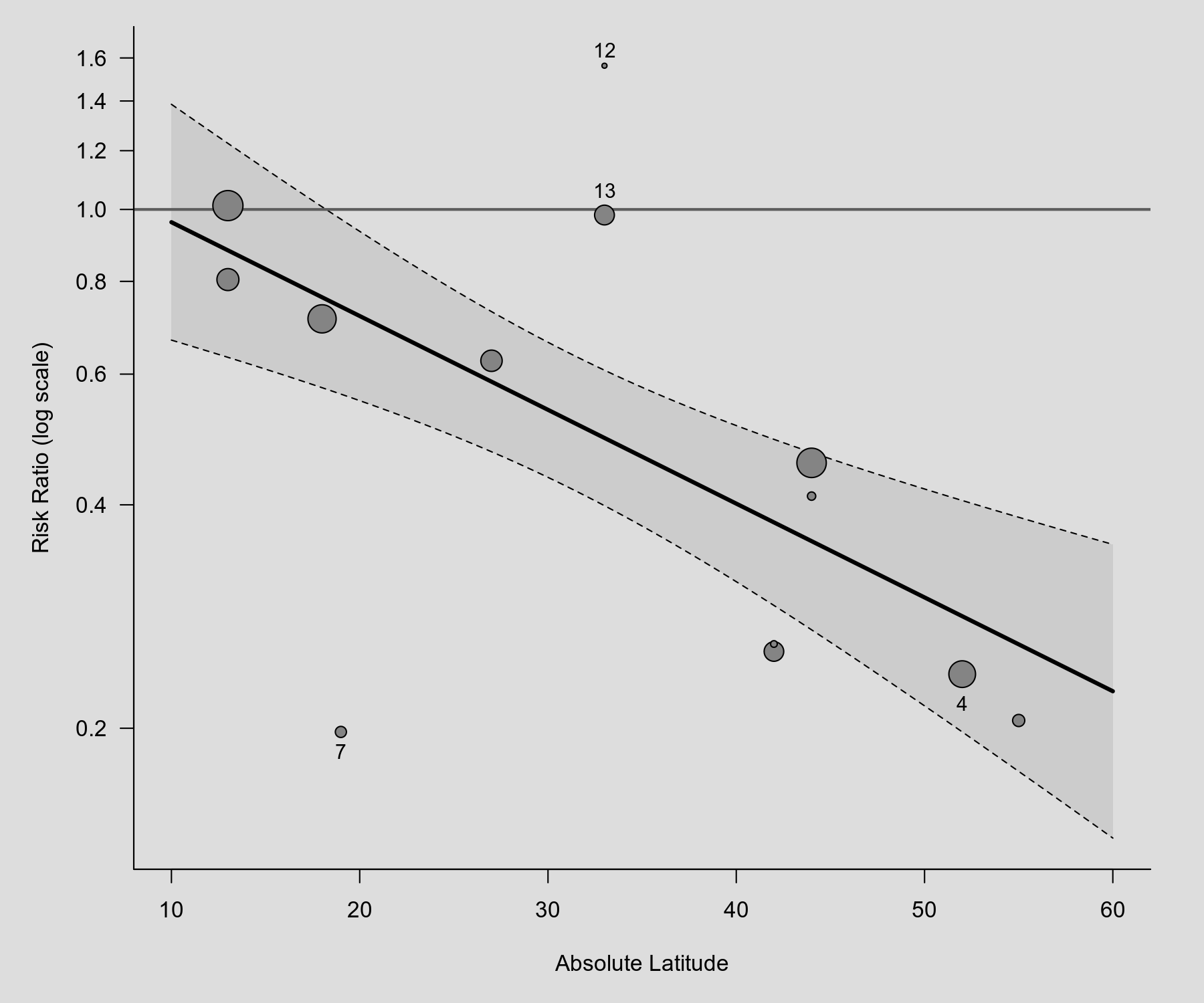#The metafor Package

A Meta-Analysis Package for R

### Sidebar

plots:meta_analytic_scatterplot

## Meta-Analytic Scatterplot

### Description

Below is an example of a scatterplot, showing the observed outcomes (risk ratios) of the individual studies plotted against a quantitative predictor (absolute latitude). The radius of the points is drawn proportional to the inverse of the standard errors (i.e., larger/more precise studies are shown as larger points). Hence, the area of the points is drawn proportional to the inverse sampling variances. Based on a mixed-effects model, the predicted average risk ratio as a function of the predictor is also added to the plot (with corresponding 95% confidence interval bounds). The scatterplot below is similar to Figure 1 in Berkey et al. (1995).

### Plot### Code

library(metafor)

### adjust margins so the space is better used
par(mar=c(5,5,1,2))

### calculate (log) risk ratios and corresponding sampling variances
dat <- escalc(measure="RR", ai=tpos, bi=tneg, ci=cpos, di=cneg, data=dat.bcg)

### fit mixed-effects model with absolute latitude as predictor
res <- rma(yi, vi, mods = ~ ablat, data=dat)

### calculate predicted risk ratios for 0 to 60 degrees absolute latitude
preds <- predict(res, newmods=c(0:60), transf=exp)

### radius of points will be proportional to the inverse standard errors
### hence the area of the points will be proportional to inverse variances
size <- 1 / sqrt(dat$vi) size <- size / max(size) ### set up plot (risk ratios on y-axis, absolute latitude on x-axis) plot(NA, NA, xlim=c(10,60), ylim=c(0.2,1.6), xlab="Absolute Latitude", ylab="Risk Ratio", las=1, bty="l", log="y") ### add points symbols(dat$ablat, exp(dat$yi), circles=size, inches=FALSE, add=TRUE, bg="black") ### add predicted values (and corresponding CI bounds) lines(0:60, preds$pred)
lines(0:60, preds$ci.lb, lty="dashed") lines(0:60, preds$ci.ub, lty="dashed")

### dotted line at RR=1 (no difference between groups)
abline(h=1, lty="dotted")

### labels some points in the plot
ids <- c(4,7,12,13)
pos <- c(3,3,1,1)
text(dat$ablat[ids], exp(dat$yi)[ids], ids, cex=0.9, pos=pos)

Berkey, C. S., Hoaglin, D. C., Mosteller, F., & Colditz, G. A. (1995). A random-effects regression model for meta-analysis. Statistics in Medicine, 14(4), 395–411.

### Page Tools